### 5. GAUSSIAN DISTRIBUTIONS

As a first application of the maximum-likelihood method, we consider the example of the measurement of a physical parameter0, where x is the result of a particular type of measurement that is known to have a measuring error. Then if x is Gaussian-distributed, the distribution function is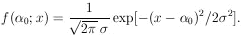For a set of N measurements xi, each with its own measurement errori the likelihood function is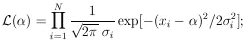then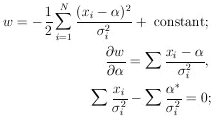(5)

The maximum-likelihood solution is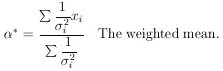(6)

Note that the measurements must be weighted according to the inverse squares of their errors. When all the measuring errors are the same we have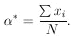Next we consider the accuracy of this determination.